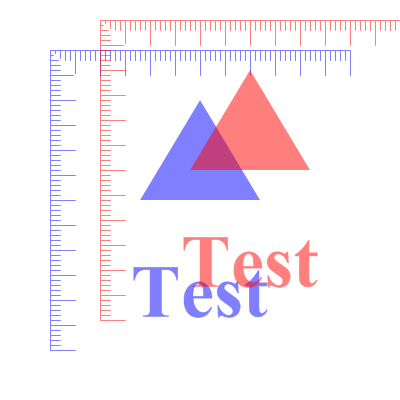TranslateCoordinates()

Syntax

TranslateCoordinates(x.d, y.d [, System])
Description
Move the origin of the vector drawing coordinate system. The move will be applied along the x/y axis of the current coordinate system. All future drawing operations will be relative to the new origin.

Parameters

 x.d, y.d Specifies the amount to move the coordinate origin along the x/y axis. System (optional) Specifies the coordinate system to change. This can be one of the following values: ``` #PB_Coordinate_User : Change the coordinate system for points in the drawing path (default) #PB_Coordinate_Source: Change the coordinate system for the vector drawing source ```

Return value

None.

Remarks

See the vectordrawing overview for an introduction to the different coordinate systems.

The following image demonstrates the effect of translated coordinates. The same figure is drawn twice, the original is in blue, and the version with translated coordinates is in red.Example

```  If OpenWindow(0, 0, 0, 400, 200, "VectorDrawing", #PB_Window_SystemMenu | #PB_Window_ScreenCentered)

If StartVectorDrawing(CanvasVectorOutput(0))
VectorFont(LoadFont(0, "Times New Roman", 60, #PB_Font_Bold))

VectorSourceColor(RGBA(0, 0, 255, 128))
MovePathCursor(50, 50)
DrawVectorText("Test")

TranslateCoordinates(30, 30) ; all coordinates are moved 30 pixels in each direction

VectorSourceColor(RGBA(255, 0, 0, 128))
MovePathCursor(50, 50)
DrawVectorText("Test")

StopVectorDrawing()
EndIf
EndIf
```